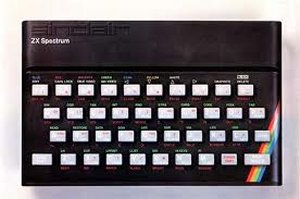Basic Commands

"Or should that be BASIC commands?"

30 LET X=X+1

This one used to drive maths people nuts. How can X be equal to X+1? Simple, it isn't. The LET bit gives it away (though LET was optional on many variants of BASIC), please Mr Computer, can you let the content of my variable X become equal to whatever is there at the moment plus 1? In this case 1+1 equals 2.

40 IF X<10 THEN GOTO 20

A bit more complicated. Firstly, the IF bit tells the computer it has a decision to make. Is the content of the variable X less than 10? If it is then do what follows after the THEN (easy to understand, IF the kettle is boiled THEN you can make a cup of tea). If the contents of X is equal or is more than 10 then do NOT do what follows the THEN but go to the next part of the line. Assuming X equals 2 at the moment then GOTO 20 simply says that instead of looking to run the next line with a number bigger than 40 then jump to line 20 and do that instead. For the smart alecs amongst you, this is known as a conditional jump since there is a condition as to whether it works or not. In our case, X is now holding the number 2 which is less than 10 so you jump back, print 2, this time, add 1 to make 3, which is still less than 10 and so on. Eventually, X will contain the number 10 in which case the condition fails and we finally move to:

50 END

And this means just what you think it means, your job is done.

Your output on screen would look like:
1
2
3
4
5
6
7
8
9
Note, no 10 printed as, in this case, it prints 9 then adds one which is no longer less than 10 so the program stops.

I still find it astonishing that people do not dabble a bit in BASIC. It is very enjoyable and is still used in things like VBA so of genuine value. If I have started you thinking then look at something like QBASIC which is nice and easy to use.